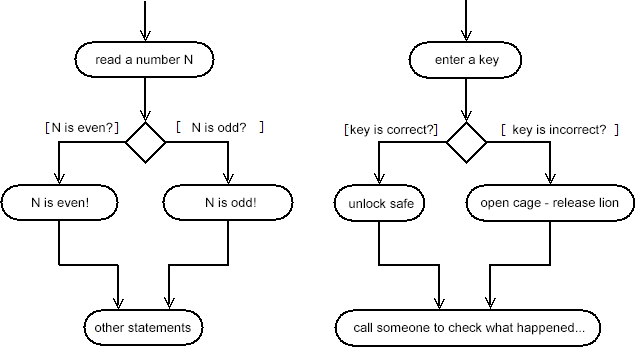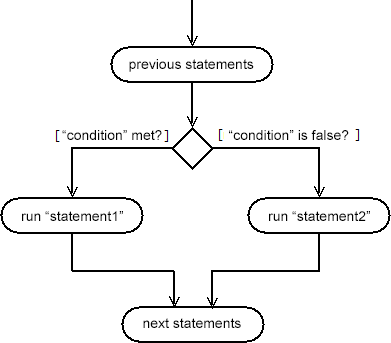## Control flow: if-else statement

### Published on 2018-04-11

Every program that we saw so far was composed of statements that are carried out sequentially (one after another). But sometimes, depending on the choice made by the user, we want our programs to perform different actions - or execute certain statements repeatedly. For example, a program can read an integer (number) from the keyboard and print whether the number is even or odd (basically, now we have two different things to print, but we only want to print one).In C++, there are several statements (called control structures) that enable us to change the program flow.

## The if-else statement

The simplest statement to enable conditional execution is the if-else statement:

if (condition)
command;

In other words, "command" is executed only if the "condition" is satisfied. Additionally, the programmer can specify a command (i.e. statement) that should be executed if the condition is not satisfied:

if (condition)
statement1;
else
statement2;Here is a program that uses if statements: the program first reads an integer, and then prints a few information about the number:

```#include <iostream>
using namespace std;

int main()
{
int n;
cin >> n;

/* only one of the 3 print statements will run */
if (n > 0)
cout << "A positive number!" << endl;

if (n < 0)
cout << "A negative number!" << endl;

if (n == 0)
cout << "The number is zero!" << endl;

/* if-else */

//if the number is divisible by 2, then it's even
if (n%2 == 0)
cout << "An even number!" << endl;
else
cout << "An odd number!" << endl;

return 0;
}
```

In C++, there is an interesting connection between integers and the values true and false (boolean). Specifically, in C++, if a condition contains an integer instead of a boolean value (true or false), then the condition will be considered unfulfilled if and only if that integer is (exactly) equal to 0. Otherwise, for any integer different than 0, the condition will be considered fulfilled:

```#include <iostream>
using namespace std;

int main()
{
int x = 4;

if (x)
{
cout << "x does not equal 0!" << endl;
}

return 0;
}
```

Because if-else is a statement in itself, it is possible to connect multiple if statements - for example, to check in which range a number belongs to:

```#include <iostream>
using namespace std;

int main()
{
int n;
cin >> n;

if (n > 0)
cout << "A positive number!" << endl;
else if (n < 0)
cout << "A negative number!" << endl;
else
cout << "The number zero!" << endl;

return 0;
}
```

C++ only performs the necessary checks to calculate the value of a condition: if we have the expression "a && b && c", and a has the value false, then the values of b and c are not important, as the expression will evaluate to false anyway (because the expression is true only if all the operands are true). With an OR expression (a || b || c) the reverse is true - if one of the operands is true, then the entire expression is true (there is no need to calculate the other values).

## Blocks

Sometimes, if a condition is fulfilled, we want to perform more than one statement. In C++, this can be done using blocks of statements.

Blocks start with the symbol '{', contain a group of statements and end with the symbol '}'. The statements in the block are separated like all other statements (using the symbol ';'). One block can be placed inside another block, which is placed inside a third block, etc...

The next program illustrates how to use blocks:

```#include <iostream>
using namespace std;

int main()
{
int n;
cin >> n;

if (n < 10)
{
cout << "The entered number is " << n << endl;
cout << "This number is less than 10." << endl;
} else
{
//a block can contain only one statement
cout << "You entered a number which is not less than 10." << endl;
}

return 0;
}
```

In all programs that we have considered so far, the variables were declared inside the main() function, before any input or output of data. But, because we have defined what blocks are, we can now mention that C++ enables us to declare variables in two ways: globally (outside of any function) and locally (in a particular function or block). Variables that are declared globally can be used at any location in the code (in any function or block). On the other hand, variables that are declared locally can be used only in the function/block in which they were defined (and after they are declared - that is, after the declaration of the variable). This, however, includes all blocks that are found inside of that function/block.

The variables declared inside the block or function may have the same name with another variable (which is defined globally or in a block). In that case, the variable declared inside (the most local variable) hides the other variables with the same name. If a local variable ("variable") hides a global variable with the same name ("variable"), we can use the operator :: to access the contents of the global variable (::variable). Although these features are offered by the C++ language, it is a good idea to try and avoid creating multiple variables with the same name (thus making the program easier to understand).

The following program illustrates these features of C++:

```#include <iostream>
using namespace std;

int variable;                           //global variable

int main()
{
int a = 10, b = 20, c = 30;         //local variables

if (b > a)
{
int d;                        //local variable
d = a+b;                      //visible only in this block

cout << d << endl;            //prints '30'
}

//the variable 'd' is not visible here

int variable;
variable = 10;                    //local variable
::variable = 100;                 //global variable

cout << variable << endl;         //prints '10'
cout << ::variable << endl;       //prints '100'

return 0;
}
```